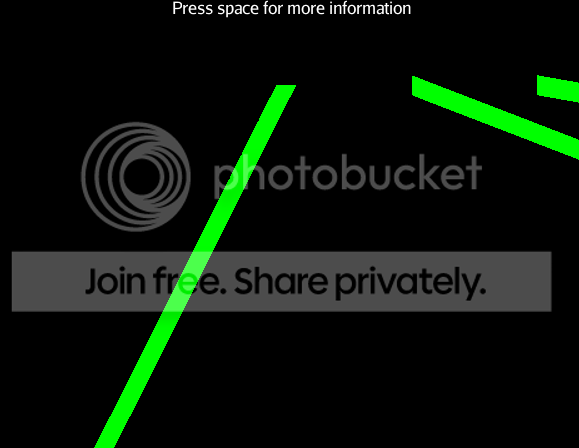# Creating a line with several points (and not just start and end)

Hello all,

How can I create a line with several points and not a lot of them with overlapping start or end.

I want to display the path of an object that moves in many directions. The only thing I got was creating a lot of lines that would overlap to look like the same line but have the feeling it’s not the way to go, can somebody point me in the right direction?

Something like this?http://www.danyrioux.com/disenthral/files/gas_giant3.png

Can’t tell from just the picture but if you’re posting it, I guess yes.

How does that translate code-wise?

Each planet has a trail showing its course (green and red lines). The stars have an orbit line (white line). The moons will soon get an orbit line too.

Those are made with lines. Is that something like this you want?

Yes, how do I make the lines?

The only thing I found was:

[java]Line line = new Line(new Vector3f(0, 5, 0), new Vector3f(5, 5, 0));[/java]

This only allows 2 points for the line and there is no method to add more points. How do I add more points?

i have seen that this uses VertexBuffers but this is the same as creating a custom mesh, no?

Well basically everything uses vertexbuffers on the lower levels.

You could use a custom mesh in line mode and “just” add all points to this, this would result in only one geometry then.

1 Like

It sounds like you want a curve.

[java]

Spline spline = new Spline(Spline.SplineType.CatmullRom, transformedPoints, 0.5f, false);

Curve curve = new Curve(spline, 10);

debugCurve = new Geometry("DebugCurve", curve);

Material mat1 = new Material(AppContext.getJme3().getAssetManager(),

mat1.setColor("Color", ColorRGBA.Blue);

debugCurve.setMaterial(mat1);

cardList.attachChild(debugCurve);

[/java]

Where transformedPoints is an array of Vector3f.

1 Like

Sad news is that I now remember to have changed from lines to something else… But here it is anyway if you want it.

This class will make a curved line or a complete circle depending on the parameters. Play with it to get what you want.

Hopefully that’ll help, if not, sorry.The class:

[java]

/**

*

*/

public final class OrbitTrail extends Mesh {

private final int samples = 128;

private final static int zSamples = 1;

public OrbitTrail(float radius, float lineWidth, float angle) {

ShortBuffer ib = BufferUtils.createShortBuffer(datapoints * 4 + zSamples * datapoints * 2);

setBuffer(Type.Index, 2, ib);

int curNum = 0;

for (int j = 0; j < zSamples; j++) {

for (int i = curNum, k = curNum + samples - 1; i < k; i++) {

ib.put((short) i).put((short) (i + 1));

}

curNum += datapoints;

}

setMode(Mode.Lines);

setLineWidth(lineWidth);

updateBound();

updateCounts();

}

public int updatePositions(float radius, float angle) {

VertexBuffer pvb = getBuffer(Type.Position);

FloatBuffer pb;

if (pvb == null) {

pvb = new VertexBuffer(Type.Position);

pb = BufferUtils.createVector3Buffer(samples);

pvb.setupData(Usage.Dynamic, 3, Format.Float, pb);

setBuffer(pvb);

} else {

pb = (FloatBuffer) pvb.getData();

}

pb.rewind();

float rate = FastMath.DEG_TO_RAD * 45 / (float) samples;

float angle2 = 0;

for (int i = 0; i < samples; i++){

float x = radius * FastMath.cos(angle + angle2);

float y = radius * FastMath.sin(angle + angle2);

pb.put(x).put(0).put(y);

angle2 += rate;

}

return samples;

}

}

[/java]

It’s used this way:

[java]

private Geometry drawOrbitLine(Planet planet, float radius, float angle) {

Geometry orbitLine = new Geometry(“Planet Orbit”, new OrbitTrail(radius, 2f, angle));

Material orbitMat = new Material(app.getAssetManager(), “Common/MatDefs/Misc/Unshaded.j3md”);

orbitMat.setColor(“Color”, new ColorRGBA(0f, 1f, 0f, 0.5f));

orbitLine.setMaterial(orbitMat);

return orbitLine;

}

[/java]

@zarch is it possible to set how thick the line is?

Probably, I can’t remember off hand. Check the javadocs.

I can only find a way to set width which gets me this:As you can see one of them is horizontal and the others vertical. This changes as the camera moves. Why?

Also, is there a way to change the shape to a cylinder?

Ok, so there is no way to configure this into what I want?